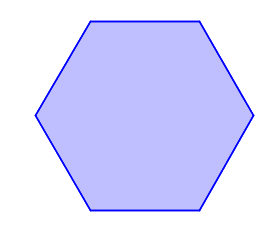### Home > CCG > Chapter 11 > Lesson 11.1.1 > Problem11-8

11-8.

Draw a hexagon on your paper.1. Do all hexagons have an interior angle sum of $720º$?

The sum of interior angles can be found by calculating $\left(n-2\right)180º$.

2. Does every hexagon have an interior angle measuring $120º$? Explain your reasoning.

No, only regular hexagons have a guarantee to having an angle with a measure of $120º$.

3. Does every hexagon have $6$ sides? Explain your reasoning.

What is the definition of a hexagon?